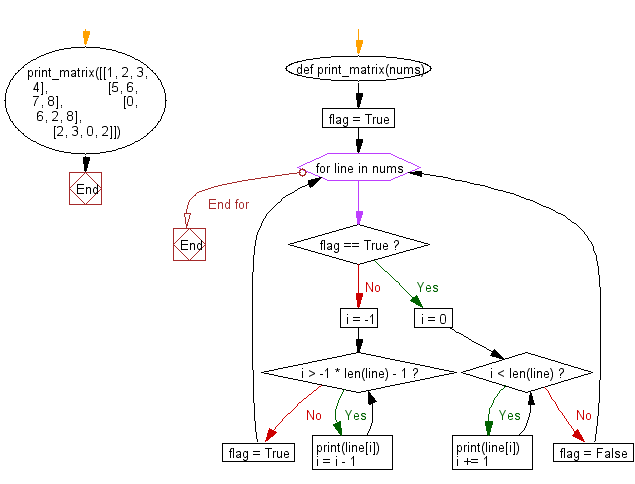﻿ Python: Print a given N by M matrix of numbers line by line in forward > backwards > forward >... order - w3resource# Python: Print a given N by M matrix of numbers line by line in forward > backwards > forward >... order

## Python Basic - 1: Exercise-78 with Solution

Write a Python program to print a given N by M matrix of numbers line by line in forward > backwards > forward >... order.

Input matrix:
[[1, 2, 3, 4],
[5, 6, 7, 8],
[0, 6, 2, 8],
[2, 3, 0, 2]]
Output:
1
2
3
4
8
7
6
5
0
6
2
8
2
0
3
2

Sample Solution:

Python Code:

``````def print_matrix(nums):
flag = True

for line in nums:

if flag == True:
i = 0
while i < len(line):
print(line[i])
i += 1
flag = False

else:
i = -1
while i > -1 * len(line) - 1:
print(line[i])
i = i - 1
flag = True
print_matrix([[1, 2, 3, 4],
[5, 6, 7, 8],
[0, 6, 2, 8],
[2, 3, 0, 2]])

``````

Sample Output:

```1
2
3
4
8
7
6
5
0
6
2
8
2
0
3
2
```

Flowchart:Python Code Editor:

Have another way to solve this solution? Contribute your code (and comments) through Disqus.

What is the difficulty level of this exercise?

Test your Programming skills with w3resource's quiz.

﻿

## Python: Tips of the Day

Iterating over dictionaries using 'for' loops:

I am a bit puzzled by the following code: d = {'x': 1, 'y': 2, 'z': 3} for key in d: print key, 'corresponds to', d[key] What I don't understand is the key portion. How does Python recognize ...

key is just a variable name.

```for key in d:
```

For Python 3.x:

```for key, value in d.items():
```

For Python 2.x:

```for key, value in d.iteritems():
```

To test for yourself, change the word key to poop.

In Python 3.x, iteritems() was replaced with simply items(), which returns a set-like view backed by the dict, like iteritems() but even better. This is also available in 2.7 as viewitems().

The operation items() will work for both 2 and 3, but in 2 it will return a list of the dictionary's (key, value) pairs, which will not reflect changes to the dict that happen after the items() call. If you want the 2.x behavior in 3.x, you can call list(d.items()).

Ref: https://bit.ly/37dm0Qo